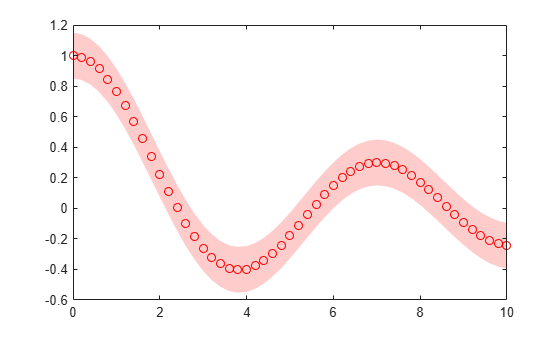Create a plot with confidence bounds using the `fill` function to draw the confidence bounds and the `plot` function to draw the data points. Use dot notation syntax `object.PropertyName` to customize the look of the plot.
```x = 0:0.2:10; y = besselj(0, x); xconf = [x x(end:-1:1)] ; yconf = [y+0.15 y(end:-1:1)-0.15]; figure p = fill(xconf,yconf,'red'); p.FaceColor = [1 0.8 0.8]; p.EdgeColor = 'none'; hold on plot(x,y,'ro') hold off```